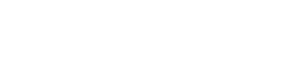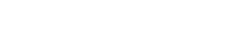# Physics Paper 2 - 2021 KCSE Prediction Questions and Answers Set 1

### Instructions to Candidates

• This paper consists of two sections A and B
• Answer all questions in section A and B
• All working must be shown in the spaces provided in this booklet.
• Mathematical tables and silent electronic calculators may be used
• Candidates should answer all the questions in English.

SECTION A– 25 MARKS

1. The figure 1 below shows a pinhole camera.Sketch rays to show the information of a diminished image in the camera. Label both the object and the image.      (2 Marks)
2. The figure 2 below shows the displacement-time graph for a certain wave.Determine the frequency of the wave.             (3 Marks)
3. A metal of resistance R1 is rated P watts, V volts while another of resistance R2 is rated 2P watts volts Determine (3 Marks)
4. A leaf electroscope A is charged and placed on the bench. Another unchanged leaf electroscope B is placed on the same bench and moved close to A until the caps touch. State and explain what is observed on the leaves A and B.        (2 Marks)
5. An unmagnetised steel rod is clamped facing North-South direction and then hammered respectively for sometime. When tested, it is found to be magnetized. Explain this observation. (2 Marks)
6. Give a reason why it is necessary to leave the caps of cells open when charging an accumulator.          (1 Mark)
7. An electromagnet is made by winding insulated copper wire on an iron core. State the two changes that could be made to increase the strength of the electromagnet.          (2 Marks)
8. Determine the speed of light in water given the speed of light in air is 3.0 x 108m/s and the refractive index of water is 1.33. (2 Marks)
9. In an x-ray tube it is observed that the intensity of x-rays increases when potential differences across the filament is increased. Explain this observation. (2 Marks)
10. The figure 3 below shows P-N junction connected to a battery. It is observed that the current in figure (a) is greater than in figure (b).Explain this observation.                (2 Marks)
11. Explain why A.C electric power is transmitted over long distance at high voltages.(1 Mark)
12. The wavelength of a radio wave is 1km. Determine its frequency. Given that V=3.0 x108
13. Identify the problem in the circuit below. (1 Mark)SECTION B – 55 MARKS

1. Figure below shows a pair of parallel plates of a capacitor connected to a battery. The upper plate is displaced slightly to the left.State with reason the effect of this movement on the capacitance.             (2 Marks)
2. Figure 6 below is electrical circuit with three capacitors A, B and C of capacitance 4.0mF, 5.0mF and 3.0mF respectively connected to 12V battery.Determine
1. the combined capacitance of the three capacitors.     (3 Marks)
2. the charge stored in capacitor A.                       (2 Marks)
3. the potential difference across the capacitor B.      (2 Marks)
1. Figure below 7 shows the path of radiation from a radioactive source. The field is perpendicular to the paper and directed out of the paper.1. State the effect of the radiation on the gas inside the Geiger-Muller tube. (1 Mark)
2. Explain how the large discharge current is created.  (2 Marks)
2. The following is a nuclear equation for a fission process resulting from the reaction of a neutron with a Uranium nucleus.1. Determine the values of x and y.       (2 Marks)
2. State the source of the energy released.          (1 Mark)
3. Explain how this reaction is made continuous in a nuclear reactor.   (2 Marks)
1. Figure 8 below shows ultra-violet light striking a polished zinc plate placed on a negatively charged gold-leaf electroscope.Explain the following observations
1. The leaf of the electroscope falls    (2 Marks)
2. When the same experiment was repeated with a positively charged electroscope the leaf did not fall. (2 Marks)
1. State two factors which determine the speed of photoelectrons emitted by a metal surface.      (2 Marks)
2. In an experiment using a photocell, U.V light of varying frequency but constant intensity was made to strike a metal surface. The maximum kinetic energy (K.Emax) of photoelectrons for each frequency, f, measured. The graph shows how K.Emax varies with f.Given that K.Emax = hf – Q, determine the value of the constants h and Q from the graph.
2. Light of frequency 5.5 x 1014HZ is made to strike a surface whose work function is 2.5ev. Show that photoelectric effect will not take place (Use the value of h from (b) (ii) above)        (4 Marks)
1. Figure 9 below shows two circuits close to each other.When the switch is closed, the galvanometer shows a reading and then returns to zero. When the switch is then opened, the galvanometer shows a reading in the opposite direction and then returns to zero. Explain these observations.          (3 Marks)
2. Explain how energy losses in a transformer are reduced by having:
1. A soft-iron core.                      (2 Marks)
2. A laminated core                     (2 Marks)
3. An ideal transformer has 2000 turns in the primary circuit and 200 turns in the secondary circuit. When primary circuit is connected to a 400V a.c. source the power delivered to a resistor in the secondary circuit is found to be 800W.
Determine the current in
1. The secondary circuit                      (3 Marks)
2. The primary circuit                         (3 Marks)
1. Figure 10 below shows a circuit in which a battery, a switch, a bulb, a resistor P, a variable resistor Q, a voltmeter V and ammeter A1 and Aof negligible resistance are connected. P has resistance of 10W. When the switch is closed voltmeter reads 1.5V.Determine
1. The current passing through P, given that the current through it is 0.1 A . (3 Marks)
2. the resistance of the bulb.                  (2 Marks)
2. The variable resistor Q is now adjusted so that a larger current flows through A2
1. State how this will affect the brightness of the bulb.         (1 Mark)## MARKING SCHEME• ✔ To read offline at any time.
• ✔ To Print at your convenience
• ✔ Share Easily with Friends / Students